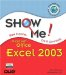# Chapter 3. Working with Formulas and Functions

 What You'll Do Create a Simple Formula Edit a Formula Understand Cell Referencing Use Absolute Cell References Use Labels for Cell References Name Cells and Ranges Simplify a Formula with Ranges Display Calculations with AutoCalculate Calculate Totals with AutoSum Correct Calculation Errors Correct Formulas Audit a Worksheet Perform Calculations Using Functions Create FunctionsShow Me Microsoft Office Excel 2003
ISBN: 0789730057
EAN: 2147483647
Year: 2002
Pages: 291